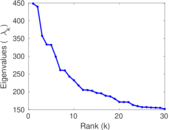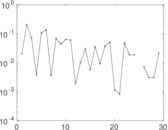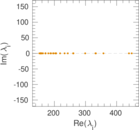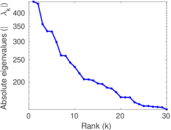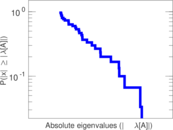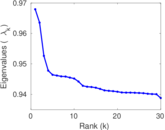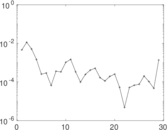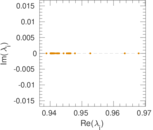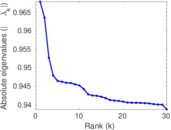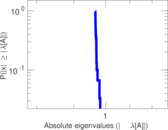This network consists of the wikilinks of the Wikipedia in the Serbian language (sr). Nodes are Wikipedia articles, and directed edges are wikilinks, i.e., hyperlinks within one wiki. In the wiki source, these are indicated with [[double brackets]]. Only pages in the article namespace are included.

 Code `Wsr` Internal name `wikipedia_link_sr` Name Wikipedia links (sr) Data source http://dumps.wikimedia.org/ AvailabilityDataset is available for download Consistency checkDataset passed all tests Category Hyperlink network Node meaning Article Edge meaning Wikilink Network format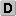Unipartite, directed Edge typeUnweighted, no multiple edges ReciprocalContains reciprocal edges Directed cyclesContains directed cycles Loops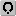Contains loops

## Statistics

 Size n = 3,175,009 Volume m = 139,586,199 Loop count l = 710 Wedge count s = 772,080,831,959 Claw count z = 42,055,975,711,170,472 Cross count x = 2.692 26 × 1021 Triangle count t = 9,089,585,833 Maximum degree dmax = 369,567 Maximum outdegree d+max = 6,444 Maximum indegree d−max = 369,553 Average degree d = 87.928 1 Fill p = 1.384 69 × 10−5 Size of LCC N = 3,174,745 Size of LSCC Ns = 617,492 Relative size of LSCC Nrs = 0.194 485 Diameter δ = 10 50-Percentile effective diameter δ0.5 = 3.639 85 90-Percentile effective diameter δ0.9 = 4.824 09 Median distance δM = 4 Mean distance δm = 4.180 98 Balanced inequality ratio P = 0.093 611 8 Outdegree balanced inequality ratio P+ = 0.090 554 6 Indegree balanced inequality ratio P− = 0.184 132 Degree assortativity ρ = −0.104 997 Degree assortativity p-value pρ = 0.000 00 Clustering coefficient c = 0.035 318 5 Directed clustering coefficient c± = 0.912 382 Operator 2-norm ν = 2,679.95 Cyclic eigenvalue π = 448.378 Reciprocity y = 0.113 018 Non-bipartivity bA = 0.068 941 3 Normalized non-bipartivity bN = 0.012 427 3

## Plots

### Degree distribution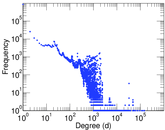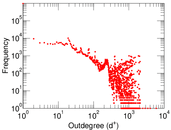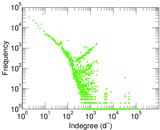### Cumulative degree distribution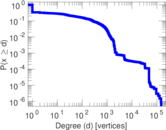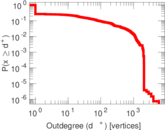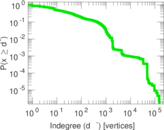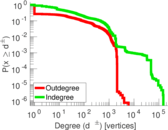### Hop distribution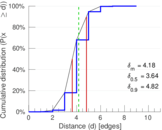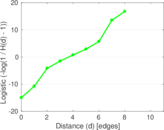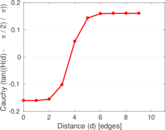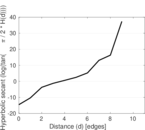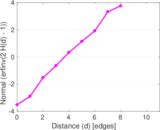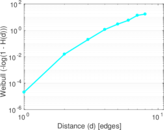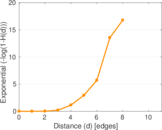### In/outdegree scatter plot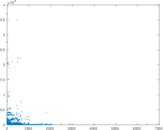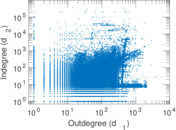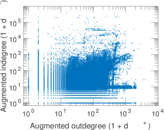### Matrix decompositions plots